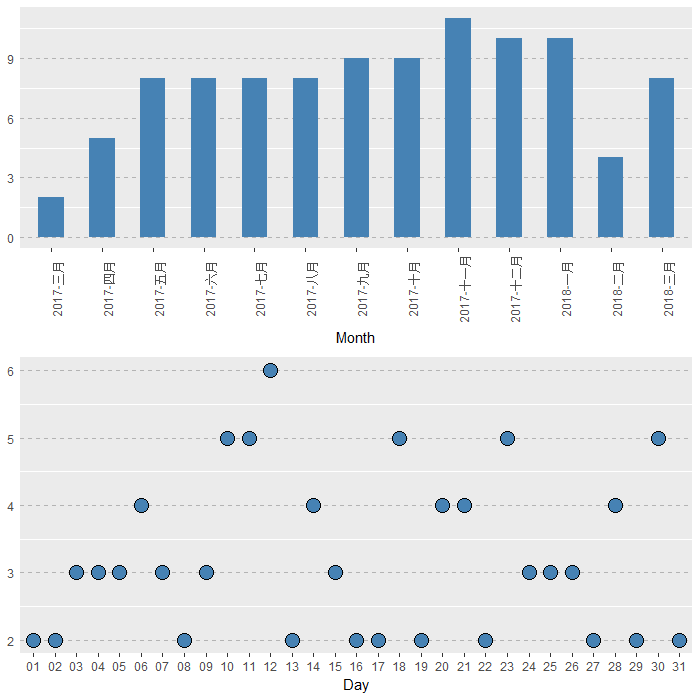## 以爬虫-纪念博客一周年

1. 首先是获取网页，用`read_html`读取网页

``````library(rvest)
url <- "http://www.bioinfo-scrounger.com"
``````
2. 接着要寻找文章名称的元素/CSS标签，这里最简单的是用`selectorgadget`谷歌插件，但是我们也要学会自己来寻找所需信息，比如从网页的源代码中可以发现，文章名称是包在元素\<a>里面的，而元素\<a>则是在类为entry-header的元素\<header>下，所以我们可以用`html_nodes`来获取这一节点的信息，`html_text`转化为文本

``````html <- html_nodes(webpage, '.entry-title a')
paper <- html_text(html)
> paper
 "R学习笔记 作图Tips"
 "修改CSS自定义wordpress主题（伪）"
 "用AnnotationHub获取非模式物种注释信息"
 "R作图 ggplot2图片的布局排版"
 "使用Shiny快速开发web程序"
 "用XML包解析uniprot的API网页"
 "Bioconductor的质谱蛋白组学数据分析"
 "Differential expression in proteomics"
 "用亚马逊的AWS来搭梯子"
 "Bioinformatics for Proteomics Data"
``````

``````library(rvest)
library(dplyr)

blog_info <- data.frame()

##因为每页只有10篇文章，所以根据URL规则循环抓取信息
for(i in 1:10){
url <- paste0("http://www.bioinfo-scrounger.com/page/", i)

##抓取文章名称
paper <- html_nodes(webpage, 'header.entry-header a') %>% html_text()

##抓取文章分类信息
class <- html_nodes(webpage, 'span.entry-categories a') %>% html_text(trim = TRUE)

##抓取文章月份/日（月份以年-月表示）
date <- html_nodes(webpage, "span.posted-on a") %>% html_text(trim = TRUE)
date_month <- unlist(lapply(date, function(x){
tmp <- strsplit(x, " ")[]
paste(tmp, tmp, sep = "-")
}))
date_day <- unlist(lapply(date, function(x){
tmp <- strsplit(x, " ")[]
sub(",", "", tmp)
}))

##抓取文章链接
href <- html_nodes(webpage, 'header.entry-header a') %>% html_attr("href")

info <- data.frame(Paper=paper, Class=class, Month=date_month, Day=date_day, URL=href, stringsAsFactors = F)
blog_info <- rbind(blog_info, info)
}

Paper    Class     Month        Day         URL
1 R学习笔记 作图Tips   R 2018-三月  21 http://www.bioinfo-scrounger.com/archives/520
2 修改CSS自定义wordpress主题（伪） 杂货笔记 2018-三月  19 http://www.bioinfo-scrounger.com/archives/516
3 用AnnotationHub获取非模式物种注释信息    Basic 2018-三月  18 http://www.bioinfo-scrounger.com/archives/512
4 R作图 ggplot2图片的布局排版        R 2018-三月  15 http://www.bioinfo-scrounger.com/archives/509
5 使用Shiny快速开发web程序        R 2018-三月  11 http://www.bioinfo-scrounger.com/archives/506
6 用XML包解析uniprot的API网页        R 2018-三月  07 http://www.bioinfo-scrounger.com/archives/504
``````

``````library(Rmisc)
library(ggplot2)

df_month <- as.data.frame(table(blog_info\$Month))
names(df_month) <- c("Month", "Num")
df_month\$Month <- factor(df_month\$Month, levels = unique(rev(blog_info\$Month)))
p1 <- ggplot(df, aes(x = Month, y = Num)) +
geom_bar(stat = "identity", fill = 'steelblue', width = 0.5) +
theme_cleveland() +
theme(
axis.text.x = element_text(angle = 90)
)
p1

df_day <- as.data.frame(table(blog_info\$Day))
names(df_day) <- c("Day", "Num")
p2 <- ggplot(df_day, aes(x = Day, y = Num)) +
geom_point(size = 5, shape = 21, fill = "steelblue") +
theme_cleveland()
p2

list_p <- list(p1, p2)
multiplot(plotlist = list_p)
````````````data_class <- table(blog_info\$Class[blog_info\$Class != "杂货笔记"]) %>% as.matrix()
data_class <- apply(data_class, 2, function(x){
x/sum(data_class[,1])
})
data_class <- data.frame("", t(data_class))

ggradar(data_class, grid.mid = 0.125, grid.max = 0.25, grid.label.size = 5,
axis.label.size = 5, group.point.size = 5)
``````